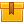# Introduction to Digital Devices (A8207)

## Prerequisites

This lesson is designed for participants familiar with basic electrical theory, electrical safety, electrical connections, electrical print reading, semiconductors, diodes, and the operating characteristics of transistors, resistors, and other basic circuit components. The ability to properly use electrical/electronic test instruments is also required.

## Description

This lesson covers how digital electronic components process and transmit information, the principles of operation of basic logic gates, and how binary numbers can be used to represent information.

## Objectives

Describe how digital electronic circuits process information. Explain the logic functions that can be performed by digital electronic circuits. Explain the truth tables associated with logic functions. Determine the logic function that is performed by a circuit. Explain an integrated circuit. Explain how the binary number system is commonly used in digital electronic circuits.

## ContentThis lesson covers how digital electronic components process and
transmit information, the principles of operation of basic logic gates,
and how binary numbers can be used to represent information.

•Introduction to Digital Devices

0
0 Reviews